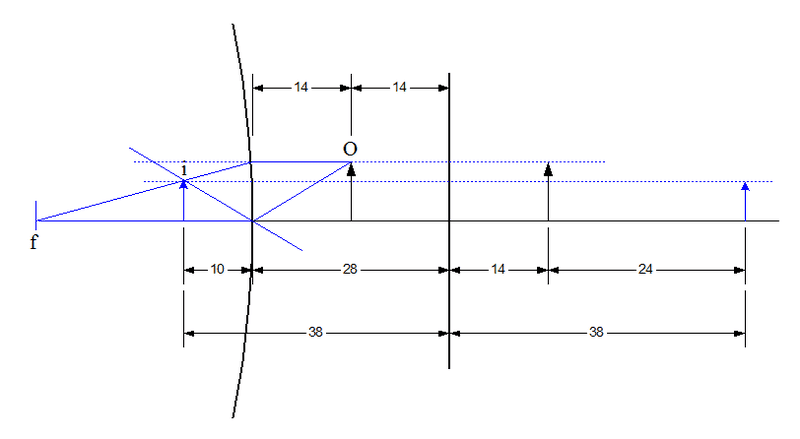# Reflection problem

• Archived
Hyperreality
A convex mirror and a plane mirror are placed facing each other and 28cm apart. Midway between them and on the principal axis of the convex mirror a small luminous object is placed. If the distance between the two images observed on looking into the plane mirror is 24 cm, calculate the radius of curvature of the convex.

I have been stuck on this problem for quite a while now.

First, theere is a image on the plane mirror, so that means the virtual image produced by the convex mirror has to be at the position of the object right?

Please give me some hints on how to tacke this problem...

## Answers and Replies

Mentor
A complete solution is offered.

The mirrors are 28 cm apart so the object being halfway between them is 14 cm from the plane mirror. Its image in the plane mirror will be 14 cm beyond the mirror. We want the the second image to be 24 cm beyond that, for a total of 38 cm behind the plane mirror.

For this to occur, its virtual image in the convex mirror must be 38 cm away from the plane mirror. The convex mirror itself already 28 cm from the plane mirror, so the virtual image must be 38 - 28 = 10 cm beyond the convex mirror.Invoke the lens / mirror equation:

##\frac{1}{O} + \frac{1}{f} = \frac{1}{i}##

Since the is a convex mirror the image is virtual so i is negative. O is negative, too, by convention. So we set:

##O = -14~cm##
##i = -10~cm##

Solve for f:

##f = \frac{O⋅i}{O - i} = -35~cm##

So the radius of curvature of the convex mirror is 35 cm.

•gracy